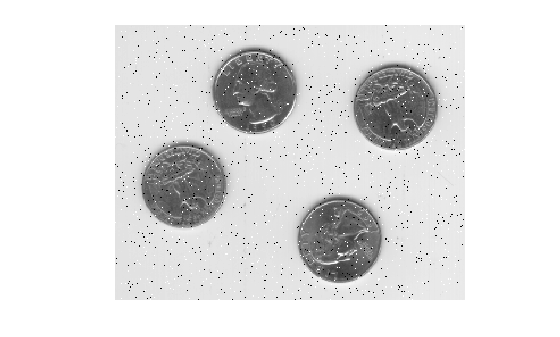# imnoise

## Syntax

``J = imnoise(I,'gaussian')``
``J = imnoise(I,'gaussian',m)``
``J = imnoise(I,'gaussian',m,var_gauss)``
``J = imnoise(I,'localvar',var_local)``
``J = imnoise(I,'localvar',intensity_map,var_local)``
``J = imnoise(I,'poisson')``
``J = imnoise(I,'salt & pepper')``
``J = imnoise(I,'salt & pepper',d)``
``J = imnoise(I,'speckle')``
``J = imnoise(I,'speckle',var_speckle)``

## Description

````J = imnoise(I,'gaussian')` adds zero-mean, Gaussian white noise with variance of 0.01 to grayscale image `I`.```
````J = imnoise(I,'gaussian',m)` adds Gaussian white noise with mean `m` and variance of 0.01.```
````J = imnoise(I,'gaussian',m,var_gauss)` adds Gaussian white noise with mean `m` and variance `var_gauss`.```
````J = imnoise(I,'localvar',var_local)` adds zero-mean, Gaussian white noise of local variance `var_local`.```
````J = imnoise(I,'localvar',intensity_map,var_local)` adds zero-mean, Gaussian white noise. The local variance of the noise, `var_local`, is a function of the image intensity values in `I`. The mapping of image intensity value to noise variance is specified by the vector `intensity_map`.```
````J = imnoise(I,'poisson')` generates Poisson noise from the data instead of adding artificial noise to the data. See Algorithms for more information.```
````J = imnoise(I,'salt & pepper')` adds salt and pepper noise, with default noise density 0.05. This affects approximately 5% of pixels.```

example

````J = imnoise(I,'salt & pepper',d)` adds salt and pepper noise, where `d` is the noise density. This affects approximately `d*numel(I)` pixels.```
````J = imnoise(I,'speckle')` adds multiplicative noise using the equation `J = I+n*I`, where `n` is uniformly distributed random noise with mean 0 and variance 0.05.```
````J = imnoise(I,'speckle',var_speckle)` adds multiplicative noise with variance `var_speckle`.```

## Examples

collapse all

Read a grayscale image and display it.

```I = imread('eight.tif'); imshow(I)```Add salt and pepper noise, with a noise density of 0.02, to the image. Display the result.

```J = imnoise(I,'salt & pepper',0.02); imshow(J)```## Input Arguments

collapse all

Grayscale image, specified as a numeric array of any dimensionality.

`imnoise` expects pixel values of data type `double` and `single` to be in the range [0, 1]. You can use the `rescale` function to adjust pixel values to the expected range. If your image is type `double` or `single` with values outside the range [0,1], then `imnoise` clips input pixel values to the range [0, 1] before adding noise.

Note

For Poisson noise, `imnoise` does not support images of data type `int16`.

Data Types: `single` | `double` | `int16` | `uint8` | `uint16`

Mean of Gaussian noise, specified as a numeric scalar.

Variance of Gaussian noise, specified as a numeric scalar.

Local variance of Gaussian noise, specified as one of the following:

Intensity values that are mapped to Gaussian noise variance, specified as a numeric vector. The values are normalized to the range [0, 1].

You can plot the functional relationship between noise variance `var_local` and image intensity using the command `plot(intensity_map,var_local)`.

Noise density for salt and pepper noise, specified as a numeric scalar. The noise is applied to approximately `d*numel(I)` pixels.

Variance of multiplicative noise, specified as a numeric scalar.

## Output Arguments

collapse all

Noisy image, returned as a numeric matrix of the same data type as input image `I`. For images of data type `double` or `single`, the `imnoise` function clips output pixel values to the range [0, 1] after adding noise.

## Algorithms

• The mean and variance parameters for `'gaussian'`, `'localvar'`, and `'speckle'` noise types are always specified as if the image were of class `double` in the range [0, 1]. If the input image is a different class, the `imnoise` function converts the image to `double`, adds noise according to the specified type and parameters, clips pixel values to the range [0, 1], and then converts the noisy image back to the same class as the input.

• The Poisson distribution depends on the data type of input image `I`:

• If `I` is double precision, then input pixel values are interpreted as means of Poisson distributions scaled up by `1e12`. For example, if an input pixel has the value `5.5e-12`, then the corresponding output pixel will be generated from a Poisson distribution with mean of 5.5 and then scaled down by `1e12`.

• If `I` is single precision, the scale factor used is `1e6`.

• If `I` is `uint8` or `uint16`, then input pixel values are used directly without scaling. For example, if a pixel in a `uint8` input has the value 10, then the corresponding output pixel will be generated from a Poisson distribution with mean 10.

• To add `'salt & pepper'` noise with density `d` to an image, `imnoise` first assigns each pixel a random probability value from a standard uniform distribution on the open interval (0, 1).

• For pixels with probability value in the range (0, `d`/2), the pixel value is set to `0`. The number of pixels that are set to `0` is approximately `d*numel(I)/2`.

• For pixels with probability value in the range [`d`/2, `d`), the pixel value is set to the maximum value of the image data type. The number of pixels that are set to the maximum value is approximately `d*numel(I)/2`.

• For pixels with probability value in the range [`d`, 1), the pixel value is unchanged.

## Version History

Introduced before R2006a

expand all